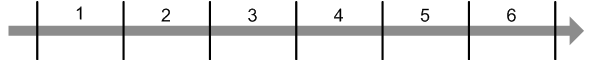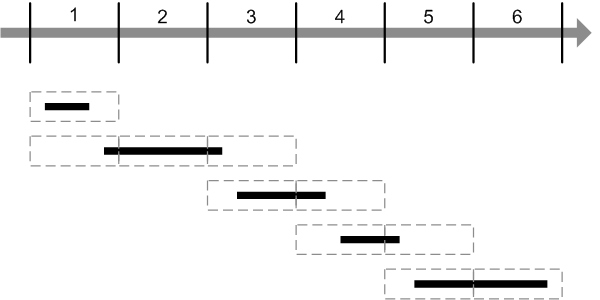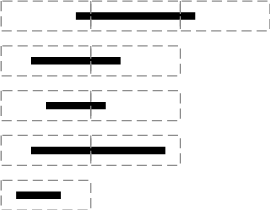# EXPLAIN EXTENDED

How to create fast database queries

## Oracle: joining timestamps and time intervals

with one comment

From Stack Overflow:

I am making an inner join of two tables.

I have many time intervals represented by `intervals` and i want to get measure data from `measures` only within those intervals. The intervals do not overlap.

Here are the table layouts:

intervals
entry_time exit_time
measures
time measure

There are 1295 records in `intervals` and about a million records in `measures`. The intervals do not overlap.

The result I want to get is a table with in the first column the measure, then the time the measure has been done, the begin/end time of the considered interval (it would be repeated for row with a time within the considered range)

How can I make this faster?

### Straight query

The query to get the results would look like this:

```SELECT  measures.measure as measure,
measures.time as time,
intervals.entry_time as entry_time,
intervals.exit_time as exit_time
FROM    intervals
JOIN    measures
ON      measures.time BETWEEN intervals.entry_time AND intervals.exit_time
ORDER BY
time ASC
```

This looks quite simple. However, choosing the execution plan can be somewhat tricky task for this query.

The table layout gives us no hint on how many rows will be returned from the join. If all intervals begin before the time of the first measure and end after the time of the last measure, then every combination of rows will be returned from both tables and the resulting recordset will in fact be a cartesian product of two tables. If the intervals are short and sparse, few or even no rows can be returned from measures.

However, we know that the intervals do not overlap. This means that each measure may belong to at most one interval and the number of records in the final resultset will be no more than the number of records in `measures`.

Since the condition we join here is a pair of inequalities, only two methods can be used by the engine to perform the join, that is `NESTED LOOPS` and `MERGE JOIN`. `HASH JOIN` which is most efficient on large tables requires an equijoin and cannot be used for this query.

`MERGE JOIN` sorts both resultsets on the columns it joins on and gradually advances the internal pointer on both sorted row sources so that the values of the columns being joined on in both tables always match. In case of an equijoin, the engine returns only the records holding the current value of the pointer; in case of a join on inequality condition, the engine returns all records greater (or less) than the current value from the corresponding table.

`MERGE JOIN`, however, can only satisfy a single inequality condition while we here have two of them on two different columns. The engine should split this condition into the pair of inequalities: the first one will be used by the join; the second one will be just filtered. The query essentially turns into this one:

```SELECT  measures.measure as measure,
measures.time as time,
intervals.entry_time as entry_time,
intervals.exit_time as exit_time
FROM    intervals
JOIN    measures
ON      measures.time &gt;= intervals.entry_time
WHERE   measures.time  &lt;= intervals.exit_time
ORDER BY
time ASC
```

Here, the `MERGE JOIN` will be using the predicate in the `ON` clause and the filtering will use the predicate in the `WHERE` clause. The predicates are symmetrical and can easily be swapped.

However, the join will have to return all records to the filtering code. And with an inequality condition like the one we see above, there will be lots of records. If we take a normal situation: the interval bounds are more or less in accordance with the dates of the first and last measure and the intervals are distributed evenly, then the `MERGE JOIN` will return `(1265 × 1,000,000) / 2 ≈ 600,000,000` records, each to be filtered on the next step.

From performance's point of view, this is hardly different from a cartesian join: in fact, it needs to process only as few as a half of the rows. These rows, however, need to be sorted beforehand (or taken from the index which is slow to traverse in its sort order), so this can actually even be slower than a cartesian join which does not require the rows to be in any certain order and can use an `INDEX FAST FULL SCAN` or a `TABLE SCAN`.

This means the only efficient way to run this query is using `NESTED LOOPS`. To benefit from this method, we should make an index on `measures.time` and convince Oracle to use it.

Let's create a sample table:

Table creation details

There are 1,500 non-overlapping intervals with random lengths from 1 to 10 days, and 1,080,000 measures. The overall spans of the intervals and the measures roughly match. `entry_time` and `exit_time` in `intervals` are indexed with `UNIQUE` indexes, and there is a composite index on `measures (time, measure)`. `measures` also contains a `stuffing` column which is a `VARCHAR2(200)` filled with asterisks. This emulates actual tables containing lots of data.

Here's a query that selects all measures within the corresponding intervals and finds the total length of their `stuffing` columns:

```SELECT  /*+ ORDERED USE_NL (i, m) */
SUM(LENGTH(stuffing))
FROM    intervals i
JOIN    measures m
ON      m.time BETWEEN entry_time AND exit_time
```
SUM(LENGTH(STUFFING))
103878600
1 row fetched in 0.0001s (10.3593s)
```SELECT STATEMENT
SORT AGGREGATE
TABLE ACCESS BY INDEX ROWID, 20091228_intervals.MEASURES
NESTED LOOPS
TABLE ACCESS FULL, 20091228_intervals.INTERVALS
INDEX RANGE SCAN, 20091228_intervals.PK_MEASURES_TIME
```

This takes a little more than 10 seconds.

The same query using `MERGE JOIN` ran for more than 10 minutes on my test machine and I had to interrupt it after that.

So the `NESTED LOOPS` is most efficient algorithm that Oracle can use for this query and it completes in 10.35 seconds.

### Interval hashing

`NESTED LOOPS` algorithm is quite efficient for a query like this, but requires traversing the index in a loop which results in a `TABLE ACCESS BY INDEX ROWID` that is needed to find the values of `stuffing`. In this very case a `HASH JOIN` with a `FULL TABLE SCAN` would be more efficient.

`HASH JOIN` requires an equality condition so we need to provide it somehow. This can be done by using a technique called interval hashing.

Here's how it works:

#### Splitting the time axis

The time axis is mapped into a set of ranges each being assigned with an ordinal number. The mapping function applied to a timestamp should uniquely define the range it belongs to. Usually this is done by splitting the time axis to the ranges of equal length and taking the integer part of the difference between the timestamp and the beginning of the first range divided by the range length:Here's an expression to do this:

```TRUNC((timestamp - TO_DATE(1, 'J')) * 2)
```

This expression splits the time axis into a set ranges each being 12 hours long.

#### Finding the ranges

For each interval, all ranges it overlaps should be found:This can be easily done by mapping the beginning and the end of each interval to their corresponding ranges and finding all the ranges between them.

This query returns the first range, the last range and total number of ranges each interval overlaps:

```SELECT  entry_range, exit_range,
exit_range - entry_range + 1 AS range_span,
entry_time, exit_time
FROM    (
SELECT  TRUNC((entry_time - TO_DATE(1, 'J')) * 2) AS entry_range,
TRUNC((exit_time - TO_DATE(1, 'J')) * 2) AS exit_range,
entry_time,
exit_time
FROM    intervals
)
```

#### Exploding the ranges

We should explode the ranges: if an interval spans `n` ranges, we should generate a recordset of `n` records for this interval, each corresponding to an individual range.

This would be an easy task if Oracle did support `generate_series` (like PostgreSQL) or `CROSS APPLY` (like SQL Server). Unfortunately, it does support neither of these, so we will have to make do with a simple join.

We should do the following:

• Find the longest interval in terms of ranges it overlaps:On the picture above, the longest interval spans 3 ranges.

Here's a query to find the interval that spans most ranges:

```WITH    splits AS
(
SELECT  /*+ MATERIALIZE */
entry_range, exit_range,
exit_range - entry_range + 1 AS range_span,
entry_time, exit_time
FROM    (
SELECT  TRUNC((entry_time - TO_DATE(1, 'J')) * 2) AS entry_range,
TRUNC((exit_time - TO_DATE(1, 'J')) * 2) AS exit_range,
entry_time,
exit_time
FROM    intervals
)
),
upper AS
(
SELECT  /*+ MATERIALIZE */
MAX(range_span) AS max_range
FROM    splits
)
SELECT  *
FROM    upper
```

I added the `MATERIALIZE` hint so that the `CTE` results are stored in the temporary tablespace and not reevaluated each time the `CTE` is called. This will be used later.

• Generate the dummy rowset of as many records as there are the ranges the longest interval overlaps:
```WITH    splits AS
(
SELECT  /*+ MATERIALIZE */
entry_range, exit_range,
exit_range - entry_range + 1 AS range_span,
entry_time, exit_time
FROM    (
SELECT  TRUNC((entry_time - TO_DATE(1, 'J')) * 2) AS entry_range,
TRUNC((exit_time - TO_DATE(1, 'J')) * 2) AS exit_range,
entry_time,
exit_time
FROM    intervals
)
),
upper AS
(
SELECT  /*+ MATERIALIZE */
MAX(range_span) AS max_range
FROM    splits
),
ranges AS
(
SELECT  /*+ MATERIALIZE */
level AS chunk
FROM    upper
CONNECT BY
level &lt;= max_range
)
SELECT  *
FROM    ranges
```
• Join the intervals to this rowset, generating one record per each range an interval overlaps:This is done using this query:

```WITH    splits AS
(
SELECT  /*+ MATERIALIZE */
entry_range, exit_range,
exit_range - entry_range + 1 AS range_span,
entry_time, exit_time
FROM    (
SELECT  TRUNC((entry_time - TO_DATE(1, 'J')) * 2) AS entry_range,
TRUNC((exit_time - TO_DATE(1, 'J')) * 2) AS exit_range,
entry_time,
exit_time
FROM    intervals
)
),
upper AS
(
SELECT  /*+ MATERIALIZE */
MAX(range_span) AS max_range
FROM    splits
),
ranges AS
(
SELECT  /*+ MATERIALIZE */
level AS chunk
FROM    upper
CONNECT BY
level &lt;= max_range
),
tiles AS
(
SELECT  /*+ MATERIALIZE USE_MERGE (r s) */
entry_range + chunk - 1 AS tile,
entry_time,
exit_time
FROM    ranges r
JOIN    splits s
ON      chunk &lt;= range_span
)
SELECT  *
FROM    tiles
```

Note that I added the hint `USE_MERGE` to the query that does the actual join. Since this is a pure inequality condition, without extra filtering, a `MERGE JOIN` is actually a best plan here.

This query returns one record for each time an interval overlaps a range. Not that though the intervals themselves do not overlap, two intervals can overlap the same range and it will be returned twice in this case.

• Now we have a recordset with the range numbers.

#### Joining on the ranges

When we have the intervals mapped into the ranges we can do just the same with the measures: map the timestamps into the ranges and join on them.

Since this would be an equijoin, a `HASH JOIN` method can be used:

View query details

We see that this query is almost 5 times as fast. Unfortunately, it returns incorrect results.

This is because joining on the ranges is not enough. The fact that both an interval and a measure overlap each other means they overlap the same range (and hence the match will be satisfied by the join). But the opposite is not true: even if an interval and a measure overlap the same range, they do not necessarily overlap each other.

This problem however, can be fixed by adding a simple condition to the `WHERE` clause of the query: it will be exactly the same condition for checking the intervals bounds against the measure time that we originally used.

Since the query returns everything we need (plus some excess data we don't need), we just need to filter the incorrect matches out.

This is essentially what the original query did, but instead of making `N×M` comparisons required by the cartesian join or half that number required by the `MERGE JOIN`, the comparisons will only need to be made inside a single range. If we divide the time axis into `R` ranges, then `R` ranges should be examined, with `(N/R)×(M/R)` comparisons within each range. This means that the total number of comparisons will be `(N×M)/R` which of course is much more efficient.

Here's the final query:

```WITH    splits AS
(
SELECT  /*+ MATERIALIZE */
entry_range, exit_range,
exit_range - entry_range + 1 AS range_span,
entry_time, exit_time
FROM    (
SELECT  TRUNC((entry_time - TO_DATE(1, 'J')) * 2) AS entry_range,
TRUNC((exit_time - TO_DATE(1, 'J')) * 2) AS exit_range,
entry_time,
exit_time
FROM    intervals
)
),
upper AS
(
SELECT  /*+ MATERIALIZE */
MAX(range_span) AS max_range
FROM    splits
),
ranges AS
(
SELECT  /*+ MATERIALIZE */
level AS chunk
FROM    upper
CONNECT BY
level &lt;= max_range
),
tiles AS
(
SELECT  /*+ MATERIALIZE USE_MERGE (r s) */
entry_range + chunk - 1 AS tile,
entry_time,
exit_time
FROM    ranges r
JOIN    splits s
ON      chunk &lt;= range_span
)
SELECT  /*+ LEADING(t) USE_HASH(m t) */
SUM(LENGTH(stuffing))
FROM    tiles t
JOIN    measures m
ON      TRUNC((m.time - TO_DATE(1, 'J')) * 2) = tile
AND m.time BETWEEN t.entry_time AND t.exit_time
```
SUM(LENGTH(STUFFING))
103878600
1 row fetched in 0.0001s (2.5312s)
```SELECT STATEMENT
TEMP TABLE TRANSFORMATION
TABLE ACCESS FULL, 20091228_intervals.INTERVALS
SORT AGGREGATE
VIEW
TABLE ACCESS FULL, SYS.SYS_TEMP_0FD9D67DD_1825AC8
CONNECT BY WITHOUT FILTERING
COUNT
VIEW
TABLE ACCESS FULL, SYS.SYS_TEMP_0FD9D67DE_1825AC8
MERGE JOIN
SORT JOIN
VIEW
TABLE ACCESS FULL, SYS.SYS_TEMP_0FD9D67DF_1825AC8
SORT JOIN
VIEW
TABLE ACCESS FULL, SYS.SYS_TEMP_0FD9D67DD_1825AC8
SORT AGGREGATE
HASH JOIN
VIEW
TABLE ACCESS FULL, SYS.SYS_TEMP_0FD9D67E0_1825AC8
TABLE ACCESS FULL, 20091228_intervals.MEASURES
```

This query completes in only 2.53 seconds.

Written by Quassnoi

December 28th, 2009 at 11:00 pm

Posted in Oracle

### One Response to 'Oracle: joining timestamps and time intervals'

1. A similar problem to yours;
Assume that we have a table:

CREATE TABLE TEST_TABLE_SIL
(
EMP_NAME VARCHAR2(10 BYTE),
ROOM INTEGER,
START_DATE DATE,
END_DATE DATE
)

And we want to calculate how many hours each room is occupied in total (for all records in the table),
multiple employees can work in the same room at the same time. I did something like that:

SELECT A.ROOM, WORKING_WITHOUT_GAP – (NVL(GAP,0)) WORKING_WITH_GAP FROM
(select ROOM,24*(max(end_date)-min(start_date)) WORKING_WITHOUT_GAP from TEST_TABLE_SIL
group by ROOM) A,
(
select ROOM,SUM(24*(MIN_DATE-MAX_DATE)) GAP from
(
select
max(end_date) over (partition by ROOM order by start_date asc) MAX_DATE,
case
when lead(start_date,1) over (partition by ROOM order by start_date asc) is null then 0
when end_date >= lead(start_date,1) over (partition by ROOM order by start_date asc)
then 0
else 1
end GAP_EXISTS,
NAME,ROOM,START_DATE,END_DATE,lead(start_date,1) over (partition by ROOM order by start_date asc) MIN_DATE
from TEST_TABLE_SIL
)
where GAP_EXISTS=1
group by ROOM)B
WHERE A.ROOM=B.ROOM(+);ilker taysi

18 Dec 14 at 12:32